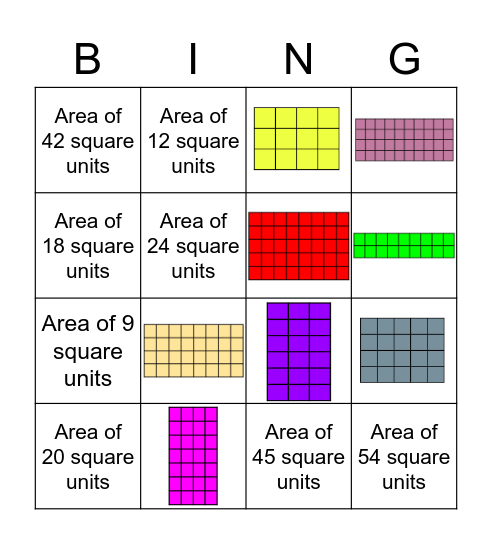# Area BingoThis bingo card has 8 images and 9 words: Area of 45 square units, Area of 20 square units, Area of 42 square units, Area of 24 square units, Area of 18 square units, Area of 12 square units, Area of 9 square units, Area of 36 square units and Area of 54 square units.

## Play Online